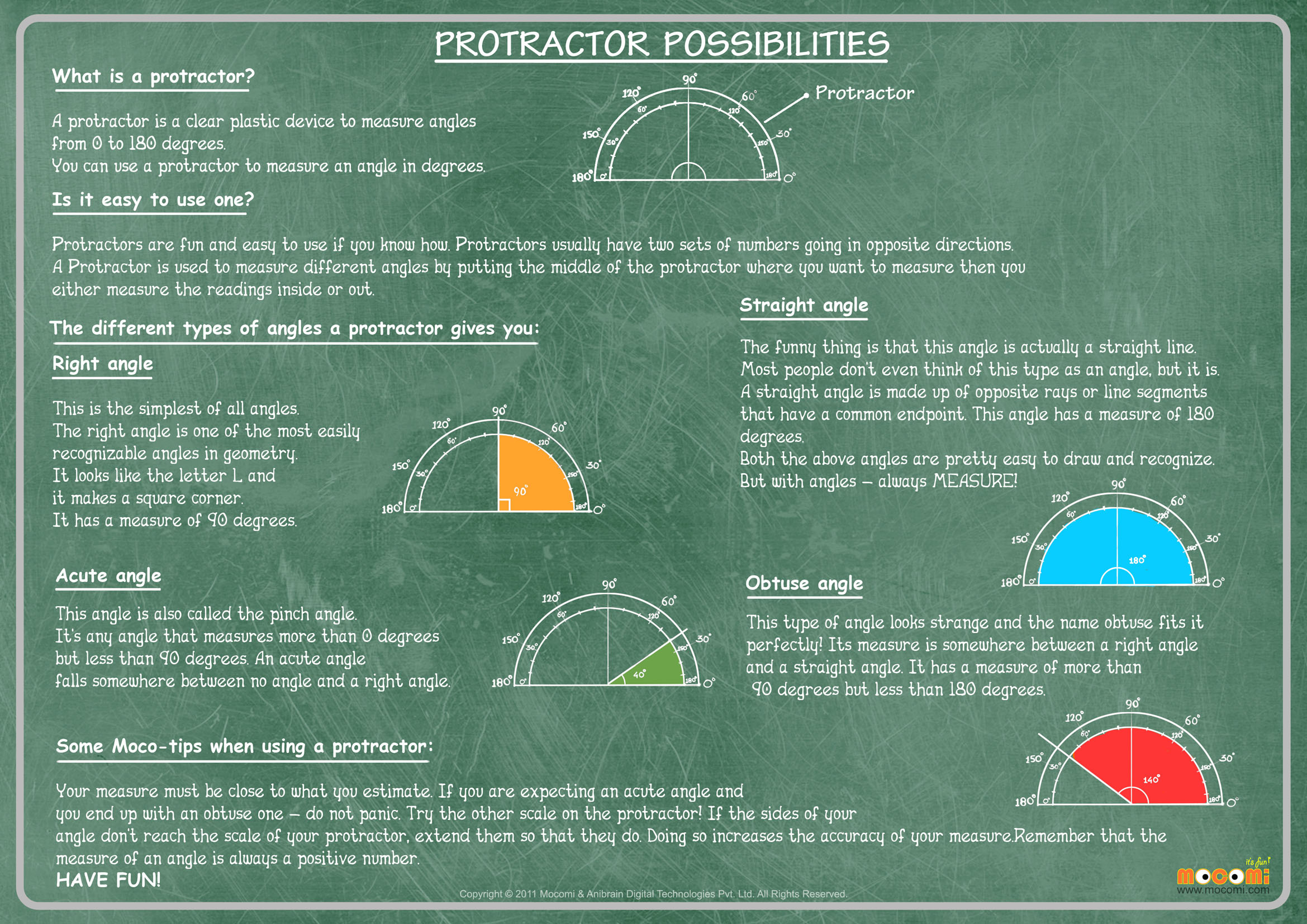• 0
• 0
• 0
• 0
• Embed CodePrevious Article
Next Article

# What is a Protractor?

Maths | 9-11 yrs | Learning Pod

What is a Protractor? – Learn about the protractor, a device used in mathematics in the area of geometry with the help of our downloadable math worksheet for kids.

Learn about the different angles a protractor gives us.

1. Right Angle
2. Acute Angle
3. Straight Angle
4. Obtuse Angle

Also get some cool Moco-tips about using a protractor.

A protractor is a clear plastic device to measure angles from 0 degrees to 90 degrees. We can use a protractor to measure an angle in degrees.

### Is it easy to use one?

Protractors are fun and easy to use if you know how. Protractors usually have two sets of numbers going in opposite directions. A protractor is sued to measure different angles by putting the middle of the protractor where you want to measure. Then you measure the reading either inside or out.

The different types of angles a protractor gives you:

### Right angle

This is the simplest of all angles. The right angle is one of the most easily recognizable angles in geometry. It looks like the letter L and it makes a square corner. It has a measure of 90 degrees.

### Acute angle

This angle is also called the pinch angle. It’s any angle that measures more than 0 degrees but less than 90 degrees. An acute angle falls somewhere between no angle and a right angle.

### Straight angle

The funny thing is that this angle is actually a straight line. Most people don’t even think of this type as an angle, but it is. A straight angle is made up of opposite rays or line segments that have a common endpoint. This angle has a measure of 180 degrees.

Both the above angles are pretty easy to draw and recognize. But with angles- always MEASURE!

### Obtuse angle

this type of angle looks strange and the name obtuse fits it perfectly! Its measure is somewhere between a right angle and a straight angle. It has a measure of more than 90 degrees but less than 180 degrees.

### Some Moco-tips when using a protractor:

Your measure must be close to what you estimate. If you are expecting an acute angle and you end up with an obtuse one- do not panic. Try the other scale on the protractor! If the sides of your angle don’t reach the scale of your protractor, extend them so that they do. Doing so increases the accuracy of your measure. Remember that the measure of your angle is always a positive number.

Have fun!

For more interesting Maths worksheets and lessons, go to : https://mocomi.com/learn/maths/

## RECOMMENDED ARTICLES

1.Mocomi Kids

@vega 😀

2.Mocomi Kids

@vega Thank you!

3.Mocomi Kids

@vega 🙂

4.vega